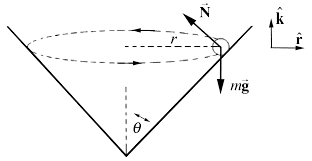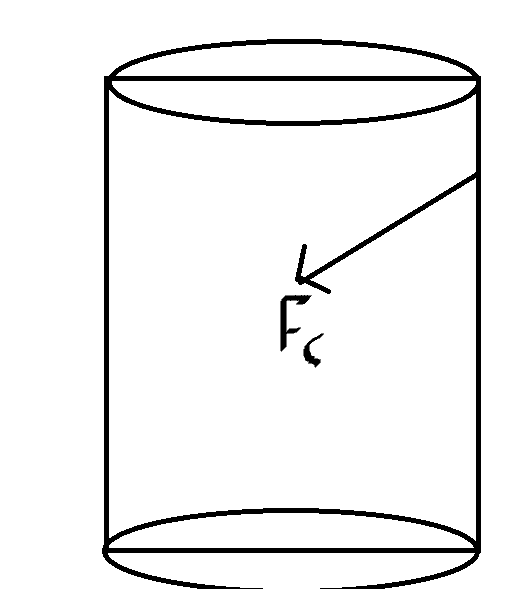# Three conceptual questions on centripetal acceleration in a cone

• aspodkfpo
Sorry, don't get it. If its velocity has a vertically downward component then by conservation of energy it is gaining KE.

#### aspodkfpo

Homework Statement
Acceleration towards central axis? Where does extra normal force come from? Motion of the ball
Relevant Equations
a = v^2/rGiven such a diagram as shown above, we know that the normal force must be mg/sintheta. How is this normal force greater than the gravitational force conceptually? Is it due to the horizontal traveling (which must have been started by someone exerting a force?) compressing the sides of the cone such that they exert a force that can maintain horizontal motion?

Now, let's assume that the net force is slightly angled downwards towards the central axis such that the ball spirals down. Is this force still regarded as a centripetal force, or is a force directed towards the central axis in a 3D plane, rather than 2D never regarded as a centripetal force?

Add a downward velocity to the ball in the diagram such that the ball goes down the cone. According to solutions, " As r decreases the component of the velocity around the cone is too large for the object to follow circular path at the lower radius and the object will begin to move outwards and rise up the cone. " How does this work? Does the normal force get larger at the bottom and why would it?

aspodkfpo said:
How is this normal force greater than the gravitational force conceptually?
If the object is maintaining its height then the normal force must supply both the vertical force to balance mg and the horizontal force to provide the centripetal acceleration.
aspodkfpo said:
assume that the net force is slightly angled downwards towards the central axis such that the ball spirals down. Is this force still regarded as a centripetal force,
Centripetal force is defined as that component of the net force which is normal to the velocity. If angled down, I would assume there is also some tangential acceleration, so the net force is more than just the centripetal force.
aspodkfpo said:
Does the normal force get larger at the bottom and why would it?
Speed is increasing and radius decreasing. Both will increase the centripetal force, and that implies an increase in normal force.

haruspex said:
If the object is maintaining its height then the normal force must supply both the vertical force to balance mg and the horizontal force to provide the centripetal acceleration.

Centripetal force is defined as that component of the net force which is normal to the velocity. If angled down, I would assume there is also some tangential acceleration, so the net force is more than just the centripetal force.

Speed is increasing and radius decreasing. Both will increase the centripetal force, and that implies an increase in normal force.

My confusion with the normal force being like this is qualitatively how it happens. If we drop a ball in normally, it will go down. So my question regarding the normal force is whether this extra normal force is caused by someone giving it speed before it goes into the cone and thereby causing greater compression along the sides of the cone.

Just realized that one of the questions doesn't really work in a cone. If we instead consider the slightly downward directed velocity in a cylinder, would this centripetal force be allowed?Last edited:
aspodkfpo said:
whether this extra normal force is caused by someone giving it speed before it goes into the cone and thereby causing greater compression along the sides of the cone.
Yes. As I posted somewhere else today, the normal force is tge minimum force required to prevent interpenetration of the bodies.
aspodkfpo said:
Which bit? If it is descending then presumably it is gaining speed.
aspodkfpo said:
If we instead consider the slightly downward directed velocity in a cylinder, would this centripetal force be allowed?
Yes, I think that is right, though perhaps not intuitive.

haruspex said:
Yes. As I posted somewhere else today, the normal force is tge minimum force required to prevent interpenetration of the bodies.

Which bit? If it is descending then presumably it is gaining speed.

Yes, I think that is right, though perhaps not intuitive.

By descending, I meant not a force, but something else bumped it and it got speed. As such, I don't see why there would be any tangential acceleration?

aspodkfpo said:
By descending, I meant not a force, but something else bumped it and it got speed. As such, I don't see why there would be any tangential acceleration?
Sorry, don't get it. If its velocity has a vertically downward component then by conservation of energy it is gaining KE.

•aspodkfpo